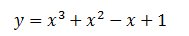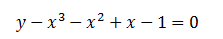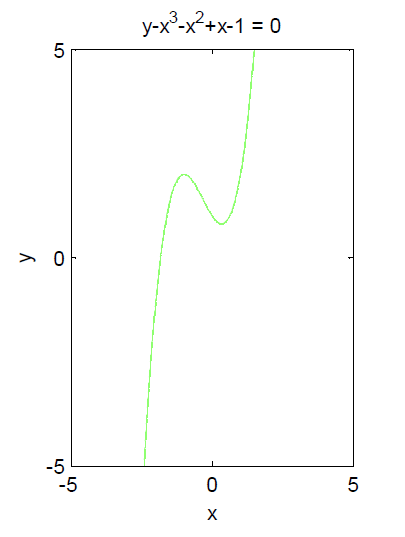Home MathLab Plotting Equations Using EZPLOT – Matlab

# Plotting Equations Using EZPLOT – Matlab

Plotting is a very important step in the study of a system since it helps understand the behavior and the restrictions of the system with less effort possible.

Plotting an equation is not always an easy task especially if you have to work out all the parameters manually, luckily nowadays there are tons of tools that help plot equations.

Matlab is the most popular tool out there when it comes to the study of the system, and in this post, we are going to work with some basic equations, Matlab, and most importantly make use of EZPLOT for plotting equations.

## Plotting equations in Matlab using EZPLOT

### Ezplot Matlab exampleTo plot this equation in Matlab using the EZPLOT, We will write the equation the following wayNow type the following code and press ENTER

`ezplot('y-x^3-x^2+x-1',[-5 5 -5 5])`

And you will obtain the following figureIf you would like to have more information about the command we just used, you can find it by using the following code

`doc ezplot`

While using the EZPLOT, the structure of the code is the following

ezplot(fun,[xmin,xmax,ymin,ymax])

Where fun is the equation, xmin and xmax are respectively the lower and the highest value of x, ymin and ymax the lowest and highest value of y. If the most interesting part of the graph your are plotting is happening in a larger interval than the one we just plotted, you may need to increase the value of xmin, xmax, ymin, and ymax.

#### Related Posts

This website uses cookies to improve your experience. We'll assume you're ok with this, but you can opt-out if you wish. Accept Read More